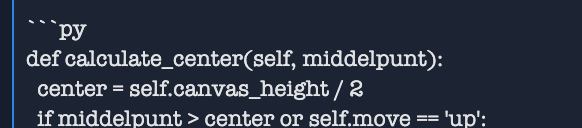Learn to Code via Tutorials on Repl.it!

← Back to all posts

# Pong AI

During the lockdown i made a pong-AI. It works by calculating the course of the ball with this code:

``````def calculate_move(self, middelpunt):
x = (bal.pos + bal.pos) / 2
y = (bal.pos + bal.pos) / 2
move_x = bal.x
move_y = bal.y
while True:
x += move_x
y += move_y
if y <= 0:
move_y = 2
if y >= self.canvas_height:
move_y = -2
if x >= self.computer_pos:
return y``````

First, this code stores the position of the ball and the speed and direction in which the ball is moving:

``````x = (bal.pos + bal.pos) / 2
y = (bal.pos + bal.pos) / 2
move_x = bal.x
move_y = bal.y``````

Then the code starts a while loop and increases the position of the ball with the speed at which the ball is moving:

``````while True:
x += move_x
y += move_y``````

When the ball is out of the playing area, it will change the y direction so that the ball comes back into the playing area:

``````if y <= 0:
move_y = 2
if y >= self.canvas_height:
move_y = -2``````

When the ball's x position is eventually past the paddle, the program returns the ball's y position, telling the paddle where to go:

``````if x >= self.computer_pos:
return y``````

When the ball goes the other way, the calculate_center() function makes the ball go back to the center and go up and down there:

``````def calculate_center(self, middelpunt):
center = self.canvas_height / 2
if middelpunt > center or self.move == 'up':
self.time += 1
self.move = 'up'
if self.time == 26:
self.move = ''
self.time = 0
return -2
elif middelpunt < center or self.move == 'down':
self.time += 1
self.move = 'down'
if self.time == 26:
self.move = ''
self.time = 0
return 2``````

For the paddle to behave a bit humanly, there is a variable self.time in the code to go up for a longer time and also for a longer time down.

##### Comments
hotnewtop
Bookie0 (5936)

You can add syntax highlighting (colors) to your code blocks by adding `py` after the e backslashes (`):``````def calculate_center(self, middelpunt):
center = self.canvas_height / 2
if middelpunt > center or self.move == 'up':
self.time += 1
self.move = 'up'
if self.time == 26:
self.move = ''
self.time = 0
return -2
elif middelpunt < center or self.move == 'down':
self.time += 1
self.move = 'down'
if self.time == 26:
self.move = ''
self.time = 0
return 2
``` :)``````